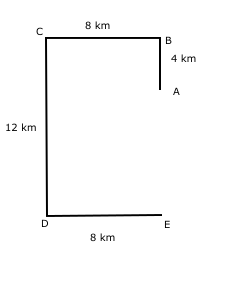0
160

Directions is one of the most important topics in the CMAT, and also it is a important section. One can utilize this article which consists of the most important questions regarding Directions. Cracku provides you with the Top 20 very Important Directions Questions for CMAT based on the questions asked in previous exam papers. Click on the link below to download the Directions Questions for CMAT PDF with detailed answers.

Question 1: In a row of children facing NORTH Ritesh is twelfth from the left end .Sudhir who is twenty second from right end is fourth to the right of Ritesh . Total how many children are there in the row?

a) 35

b) 36

c) 37

d) 34

e) None of these

Solution:

Ritesh is 12th from the left end. Sudhir is fourth to the right of Ritesh. Hence, Sudhir is 16th from the left end. And also Sudhir is 22nd from the right end. There are 11 people to the left of Ritesh, 21 people to the right of Sudhir and 3 people in between Ritesh and Sudhir. The total number of children’s in the row is: 11 + 3 + 21 + 2(including both Ritesh and Sudhir) = 37. Hence, option C is correct.

Question 2: Vikas walked 9 km towards North, took a left turn and walked 5 km and again took a left turn and walked 9 km and stopped walking. Towards which direction was he facing when he stopped walking?

a) South

b) South-West

c) South-East

d) Cannot be determined

e) None of these

Solution:

Let Vikas started from point A and head north for 9 km to reach B, then he turned left and travelled west for 5 km before reaching C, again he turned left and travelled for 9 km and finally stopped at point D.

Thus, he is facing south direction when he stopped walking.

=> Ans – (A)

Question 3: In a row of children facing North, Ritesh is twelfth from the left end. Sudhir who is twenty-second from the right end is fourth to the right of Ritesh. Total how many children are there in the row ?

a) 35

b) 36

c) 37

d) 34

e) None of these

Solution:

Ritesh’s position from left end = 12th

Sudhir is fourth to the right of Ritesh, => Sudhir’s position from left end = 12 + 4 = 16th

Also, Sudhir’s position from right end = 22nd

=> Total number of children in the row = 16 + 22 – 1

= 37

Question 4: Veena walked 5 m towards north, took a left turn and walked 7 m. She took a left turn again and walked 8 m before taking a left turn and walking 7 m. She then took a final left turn and walked 1 m before stopping. How far is Veena from the starting point?

a) 3m

b) 6 m

c) 4 m

d) 2 m

e) 7 m

Solution:

Let Veena started from point A, walked 5 m towards north and reached point B.

Then he took a left turn and reached point C after walking 7 m and then after point D after another left turn of 8 m.

His final position is point F.

Thus, distance AF = 8 – 5 – 1 = 2 m

=> Ans – (D)

Question 5: A person starts from his house and moves towards the market. He walks 40 metres towards south and takes a right turn. After walking 30 metres he takes a left turn and walks 20 metres. Finally he takes a left turn and reach the market after walking 30 metres. How far and in which direction is his house from the market ?

a) 60 metres, South

b) 60 metres, North

c) 70 metres. North

d) 70 metres, South

e) 90 metres, North

Solution:

Let the person initially be at point H and then walks 40 metres south to reach point B.

He then takes right turn to reach C which is 30 metres apart. He takes left turn and walks for 20 metres to reach point D.

Finally, he again takes a left turn for 30 metres to reach the market M.

House is in the north direction from the market, distance HM = 40 + 20 = 60 metres

=> Ans – (B)

Question 6: Point A is 30 metres to the east of point B. Point C is 10 metres to the south of Point A. Point D is 15 metres to the west of Point C. Point E is exactly in the middle of the Points D and F. Points D, E and F lie in a straight line. The length of the line DEF is 20 metres. Point F is to the north of Point D. Point G is 15 metres to the east of Point F. How far and in which direction is Point G from Point A ?

a) 10 metres, South

b) 15 metres. North

c) 10 metres, North

d) 15 metres, South

e) 10 metres, East

Solution:

Point D is 15 metres to the west of Point C and point F is to the north of Point D.

Point E is exactly in the middle of the Points D and F, => E is 10 metres to the north of D and 10 metres o the south of F.

E also lies exactly between B and A.

Point G is 15 metres to the east of Point F.

Point G is in north direction from point A, distance AG = 10 metres

=> Ans – (C)

Question 7: A person starts walking from his home towards his friend’s place. He walks for 25m towards West. He takes a 90° right turn and walks for 20m. He again takes a 90° right turn, and walks for 10m. He then walks for another 10m after taking a 90° left turn. Turning 90° towards his right, he walks for 15m to reach his friend’s place. How far and in which direction is the friend’s place from his home ?

a) 30m towards East

b) 30m towards North

c) 40m towards South

d) 30m towards South

e) 40m towards North

Solution:

He starts from point H and walks for 25m towards West and reaches G. He takes a 90° right turn and walks for 20m to reach C.

He again takes a 90° right turn, and walks for 10m and reaches point D. He then walks for another 10m after taking a 90° left turn towards North to reach point E.

Finally turning 90° towards his right, he walks for 15m to reach his friend’s place F.

His friend’s house is in the north direction from his house. Distance = 20 + 10 = 30 m

=> Ans – (B)

Question 8: Point A is 30m to the South of point B. Point C is 20 m to the East of point A. Point D is 15m to the south of point C. Point D is exactly midway between points E and F
in such a manner that point E, D and F form a horizontal straight line of 40m. Point E is to west of point D. How far and in which direction is point E from point B ?

a) 45m towards South

b) 25 m towards south

c) 3ern towards west

d) 35m towards north

e) 45 m towards north

Solution:

Point A is 30 m to the South of point B and Point C is 20 m to the East of point A. Point D is 15m to the south of point C.

Point D is exactly midway between points E and F and distance of the line is 40 m.

Point E is to west of point D, => F is to the east of D and distance between ED and DF is 20 m.

Point E is in south direction from point B. Distance BE = 30 + 15 = 45 m

=> Ans – (A)

Question 9: Town D is 13 km towards the East of town A. A bus starts from town A, travels 8 km towards West and takes a right turn. After taking the right turn, it travels 5 km and reaches town B. From town B the bus takes a right turn again, travels 21 km and stops. How far and towards which direction must the bus travel to reach town D ?

a) 13 km towards South

b) 5 km towards West

c) 21 km towards South

d) 5 km towards South

e) None of these

Solution:

The bus travels 8 km towards west from A and reaches point C, then it takes right turn and travel 5 km to reach point B.

Finally, the bus travelled east for 21 km and reached point E.

The bus must travel towards south direction to reach point D, distance = DE = 5 km.

=> Ans – (D)

Question 10: Town D is to the West of town M. Town R is to the South of town D. Town K is to the East of town R. Town K is towards which direction of town D ?

a) South

b) East

c) North-East

d) South-East

e) None of these

Solution:

Town R is to the South of town D. Town K is to the East of town R,

=> Town K is towards south-east of town D.

=> Ans – (D)

Question 11: W walked 40 metres towards West. took a left turn and walked 30 metres. He then took a right turn and walked 20 metres. He again took a right turn and walked 30 metres. How far was he from the starting point ?

a) 70 metres

b) 60 metres

c) 90 metres

d) Cannot be determined

e) None of these

Solution:

W started from A and walked 40 metres towards West to reach point B. He took a left turn and walked 30 metres and reached C.

He then took a right turn and walked 20 metres to reach D and finally took a right turn and walked 30 metres reaching point E.

Distance between his final and starting position, AE = 40 + 20 = 60 metres

=> Ans – (B)

Question 12: In a row of twenty-five children facing South R is sixteenth from the right end and B is eighteenth from the left end. How many children are there between R and B?

a) 2

b) 3

c) 4

e) None of these

Solution:

Total number of children in the row = 25

Position of R from right end = 16th

Position of B from left end = 18th

=> Position of B from right end = (25 – 18) + 1 = 8th

$\therefore$ Number of children between R and B = (16 – 8) – 1 = 7

=> Ans – (E)

Question 13: Manoj started from Point A and walked 18 m towards North. He then took two consecutive left turns and walked 5 m after taking each turn, He again took a left turn, walked 5 m and reached point B. How far is point A from point B ?

a) 15 m

b) 5 m

c) 13 m

d) 23 m

e) Cannot be determined

Solution:

After walking 18m towards north from point A, lets say he reached point C. => AC = 18

After taking two consecutive left turns of 5m each, he reached point B.

=> CBA are collinear in that order.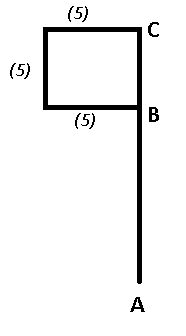=> BC = 5m

Now, distance between A and B = 18-5 = 13m

Question 14: Anup starts from point A and travels towards north for 8 km and then he turns to his left and travels for 15 km to reach point B.
What is the distance between A and B and the direction to which Anup is finally facing?

a) 17 km, West.

b) 23 km, East.

c) 19 km, West.

d) 21 km, East.

e) None of these

Solution:

Based on the given information, the path travelled by Anup could be as below.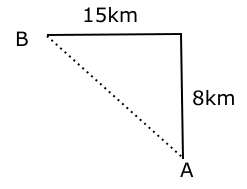Distance between A and B = $\sqrt{8^2 + 15^2}$ = 17 km.

Anup faces west at point B.

So the correct option to choose is A.

Question 15: Prajwal starts from point P and travels towards the east for 5km and then he turns right to travel for another 5km and then he turns to his left and reaches point B after travelling 7km.
What is the distance between P and B?

a) 12 km

b) 13 km

c) 9 km

d) 11 km

e) None of these

Solution:

Based on the given information, the path travelled by Prajwal could be as below.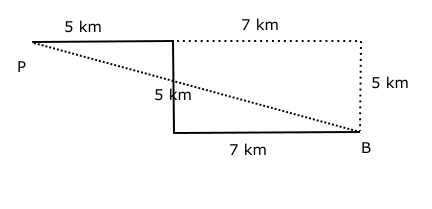The distance between P and B = $\sqrt{5^{2} + 12^{2}}$ = 13 km.

So the correct option to choose is B-13 km.

Question 16: Karan starts from point A and travels towards north for 5 km. He then takes a right turn and travels for 7 km. He then takes a left turn and travels another 7 km. Finally he takes another left turn and travels for 2 km arriving at a point B.
What is the distance between point A and B?

a) 13 km

b) 12.33 km

c) 12 km

d) 10 km

e) None of these

Solution:

Based on the given information, the path travelled by karan could be derived as below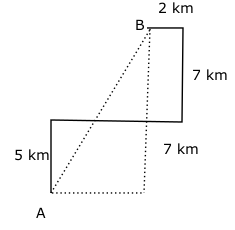Therefore the distance between A and B = $\sqrt{12^{2} + 5^{2}}$ = 13

So the correct option to choose is A – 13 km.

Question 17: Ganesh starts from point A and travels towards the east for 36 km to reach point B, at B he takes a right turn and travels for 77 km to reach point C.
What is the distance between the points A and C?

a) 75

b) 89

c) 86

d) 85

e) none of these

Solution:

Based on the given information, the path travelled by Ravi could be as below.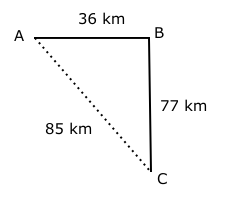Distance between A and C = $\sqrt{77^{2} + 36^{2}}$

So the correct option to choose is C – 85

Question 18: Sita starts from point A and travels towards north for 2 km, then she takes a turn of 60° to her right and travels for another 2 km, then she takes a turn of 120° to her right and travels for another 2 km. Finally, she takes a turn of 135° to her left.
What is the direction she is finally facing?

a) North

b) South -west

c) North-east

d) East

e) Cannot be determined

Solution:

Based on the given information, the path travelled by sita could be as below.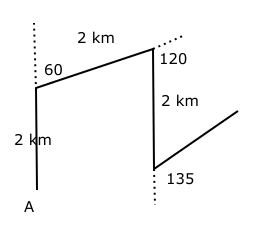Therefore the direction she finally faces is North-east.

Question 19: Abhishek starts from the point A and rides towards north for 5 km and then he turns right and rides for 10 km to take a left turn and then he rides for 6 km to take another left turn, then he rides for another 7 km to take another left turn and he finally stops at a point after travelling 11 km.
What is the final position of Abhishek with respect to point A?

a) 3 km towards the east

b) 5 km towards the west

c) 7 km towards the west

d) 5 km towards the east.

e) Cannot be determined.

Solution:

Based on the given information, the path travelled by abhishek could be as below.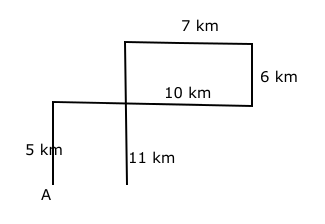==> The final point is 3 km towards the east of point A.

So the correct option to choose is A – 3km towards the east.

Question 20: Gautham starts from point A and rides towards north for 4 kms to reach B, then he takes left turn and travel for 8 kms to reach point C and then he takes another left turn and travel for 12 kms to reach point D. Finally he takes another left turn and travel for another 8kms to reach point E. What is the current position of Gautham with respect to the point A?

a) 8 kms towards east

b) 12 kms towards south

c) 12 kms towards west

d) 8 kms towards south

e) Cannot be determined